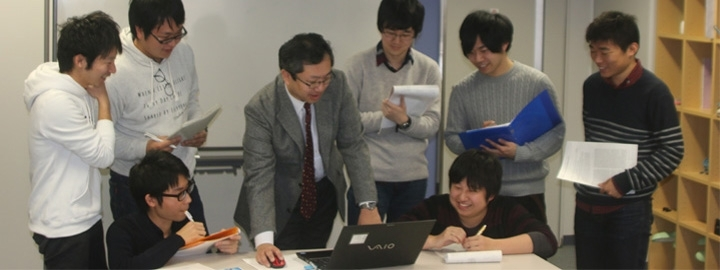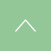# Department of Intelligence Science and Engineering

## Applied Mathematics and Physics Division### Overview

In addition to developing mathematical engineers who can work with phenomena from microscales to macroscales based on conventional mathematics, physics, and programming, the department educates and conducts research in the fields of advanced mathematical modeling, designing new materials by computer simulation, applying basic and developmental theories to elucidate physical phenomena with in-depth understandings of mathematics, physics, and computer science. Our goal is also to develop engineers who are capable of addressing information technologies and robotics utilized in real-world industries.

### Laboratory introduction

For faculty member details, please see the staff introductions.

Laboratory Theory of physical properties Professor *
Laboratory Theory of differential equations Professor USAMI Hiroyuki Employing techniques of pure mathematics and theoretical mathematics, our laboratory extensively considers qualitative theories of functional equations, primarily including ordinary differential equations and elliptic partial differential equations, as well as various orders of difference equations. In particular, we aim to elucidate the nature of asymptotic behavior, stability, and fluctuation of solutions.
Laboratory Theory of differential Equations Assistant professor Naoto Kajiwara In our laboratory, we study the solvability of differential equations and the behavior of their solutions. I would like to obtain quantitative and qualitative information from equations using functional analytic methods and real analytic methods. I am also interested in abstract theories such as analytic semigroup theory and maximal regularity theory.
Laboratory Dynamical systems theory Professor KAMEYAMA Atsushi In the field of mathematics referred to as dynamical systems, our laboratory conducts research especially of complex dynamics, reduced-dimensional systems, and fractals from topological and combinatorial viewpoints. Currently, we are engaged in coding with Julia sets of symbolic dynamics and analyzing parameter space dynamics of rational functions using this coding.
Laboratory Computational physics engineering Professor TERAO Takamichi Our laboratory conducts research of the development of computer simulation techniques suitable for metamaterial composites and their applications. From the viewpoint of computational science, we are engaged in the prediction of physical properties in microparticle-related systems, and molecular simulations to clarify the order of formation.
Laboratory Algebraic number theory Professor KOBAYASHI Takako Our research field is algebraic number theory. We study class field theory, and in particular, higher-order class field theory, class number, and special functions. For example, we focus on: expression of higher-order local class field theory with the K-group of Galois modules; expression of cyclotomic fields with Maillet and Demyanenko determinants; functional equations and special values of various Epstein zeta functions with respect to the series of coefficients; properties and relational expressions of Bernoulli and Clausen functions; and, multiplexing of these functions. These studies are based on prime numbers. The study of prime number traces back to the era of ancient Greece. We are continuing this study to touch upon the profundity of prime numbers.
Laboratory Partial differential equations, plasmas, reaction-diffusion equations Associate professor KONDO Shintaro My specialty is the mathematical analyses of nonlinear partial differential equations. Our laboratory has been conducting the study of mathematical analyses of partial differential equations and ordinary differential equations that describe the turbulent flow phenomena of plasma fusion. Recently, we are engaged in research to understand biological issues with the use of reaction-diffusion equations. Also, we have been working to elucidate misperception phenomena through modeling and mathematical analyses of a hierarchical model of the retina based on control mechanisms by "darkening bright areas and brightening dark areas."
Laboratory Quantum many-body theory, nuclear structure theory Associate professor SAKAMOTO Hideo Our laboratory performs research of issues involving quantum many-body physics in order to elucidate the mechanisms that express collective motions in many-body systems at the microscopic level based on constituent particles and their interactions. Focusing on the role of collective motion to restore symmetry breaking caused by mean-field based approaches and the restoration of symmetry breaking by introducing self-consistent effective interactions and the many-body force, we are working on construction and analyses of mathematical models of quantum many-body systems, analyses of nuclear spectroscopy experimental data, and development of methods for many-body issues such as the boson-expansion method, and analysis programs.
Laboratory Space science (radio astronomy) Associate professor TAKABA Hiroshi Assistant professor SUDO Hiroshi Assistant professor SANO Hidetoshi Our laboratory conducts observational research of radio astronomy. We are working primarily to observe water maser radio waves around a fixed star using an 11m radio telescope installed at the campus of Gifu University, software development for data analyses, and the study of black holes in galactic nuclei with the VLBI (very long baseline interferometry) observation method.
Laboratory Nitta laboratory Professor NITTA Takahiro Our laboratory studies: (1) biomolecular motors, and (2) sliding friction. (1) With the goal of applying a biomolecular motor to biosensors and microrobots, and understanding biological systems through comparisons with artifacts such as machines, we are currently engaged in experimentation and computer simulations. (2) With the goal of understanding sliding friction on a macroscopic scale, we are particularly focusing on developing a method for in-situ observations of friction interfaces.
Laboratory Additive processes Associate professor YAMAMURO Kouji Our research field covers stochastic processes, and in particular, additive processes based on probability theory from the perspective of measurement theory. Additive processes are rich mathematical objects, and typically include Brownian motion, Poisson processes, and stability processes. The study of additive processes assumes a basic role in the study of stochastic processes.
Laboratory Linear ordinary differential equations Assistant professor ASAKAWA Hidekazu Semi-linear elliptical equations appear in various fields of science, such as astrophysics, quantum mechanics, theories of reaction-diffusion systems, and mathematical biology. Our primary interest is the understanding of solutions for semi-linear elliptic equations, through an approach that is mainly based on linear differential equations and the theory of regular variations.